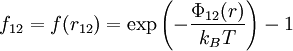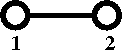# Difference between revisions of "Mayer f-function"

The Mayer f-function, or f-bond is defined as:$f_{12}=f(r_{12})= \exp\left(-\frac{\Phi_{12}(r)}{k_BT}\right) -1$

where

•$k_B$ is the Boltzmann constant.
•$T$ is the temperature.
•$\Phi_{12}(r)$ is the intermolecular pair potential.

Diagrammatically the Mayer f-function is written as